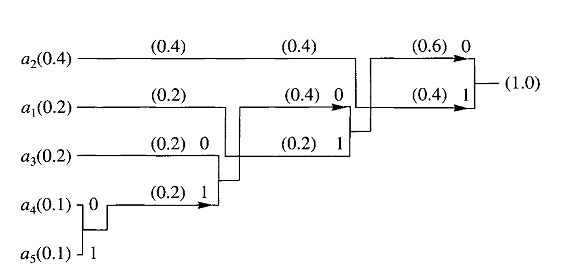1# DESC

## Lossless compression

Lossless compression schemes compress the data without loss of information, and the original data can be recovered exactly from the compressed data.

 The entropy is a measure of the average number of binary symbols needed to code the output of the source
 Entropy presented mathematically by the equation H = P(A)I(A) P(A) : Presents the probability of event A occurrence I(A) : Presents the amount of information included in event A I(A) = -LOGb P(A) H = SUM(-P(Ai) * LOGb P(Ai))
 The best that a lossless compression scheme can do is to encode the output of a source with an average number of bits equal to the entropy of the source.
More
0

# Huffman

 The Huffman procedure is based on two observations regarding optimum prefix codes.
 I. In an optimum code, symbols that occur more frequently (have a higher probability of occurrence) will have shorter codewords than symbols that occur less frequently.
 2. In an optimum code, the two symbols that occur least frequently will have the same length.
 For the second observation Suppose an optimum code e exists in which the two codewords corresponding to the two least probable symbols do not have the same length. Suppose the longer codeword is k bits longer than the shorter codeword. Because this is a prefix code, the shorter codeword cannot be a prefix of the longer codeword. This means that even if we drop the last k bits of the longer codeword, the two codewords would still be distinct. As these codewords correspond to the least probable symbols in the alphabet, no other codeword can be longer than these codewords; therefore, there is no danger that the shortened codeword would become the prefix of some other codeword. Furthermore, by dropping these k bits we obtain a new code that has a shorter average length than e. But this violates our initial contention that e is an optimal code. Therefore, for an optimal code the second observation also holds true.

## EXDesign a Huffman code Consider this Sequence "rrmmmmklnn"

 Let a1 = r a2 = m a3= n a4=k a5= l P(a1) = 0.2 P(a2) = 0.4 P(a3) = 0.2 P(a4) = 0.1 P(a5) = 0.1
More
images\4\3010\HUFFMANEx.jpg
images\4\3010\HUFFMANEx.jpg
1

# Computer Model

## UMLClass Diagram

## Flow ChartFlow Chart

More
images\4\3009\HUFFMANUmlSmall.jpg;images\4\3007\HUFFMANChartMainSmall.jpg;images\4\3008\HUFFMANChartSetCodeStringSmall.jpg;images\4\3006\HUFFMANChartInsertToBinaryTreeSmall.jpg
images\4\3009\HUFFMANUML.jpg;images\4\3007\HUFFMANChartMain.jpg;images\4\3008\HUFFMANChartSetCodeString.jpg;images\4\3006\HUFFMANChartInsertToBinaryTree.jpg
2

# Source Code

## Edited By

Eng : Sameh Mohamed Dabour

## Source Code

Uncompress file and run using Microsoft Visual Studio

Huffman.zip
More
0

# DESC

## Lossless compression

Lossless compression schemes compress the data without loss of information, and the original data can be recovered exactly from the compressed data.

 The entropy is a measure of the average number of binary symbols needed to code the output of the source
 Entropy presented mathematically by the equation H = P(A)I(A) P(A) : Presents the probability of event A occurrence I(A) : Presents the amount of information included in event A I(A) = -LOGb P(A) H = SUM(-P(Ai) * LOGb P(Ai))
 The best that a lossless compression scheme can do is to encode the output of a source with an average number of bits equal to the entropy of the source.
More